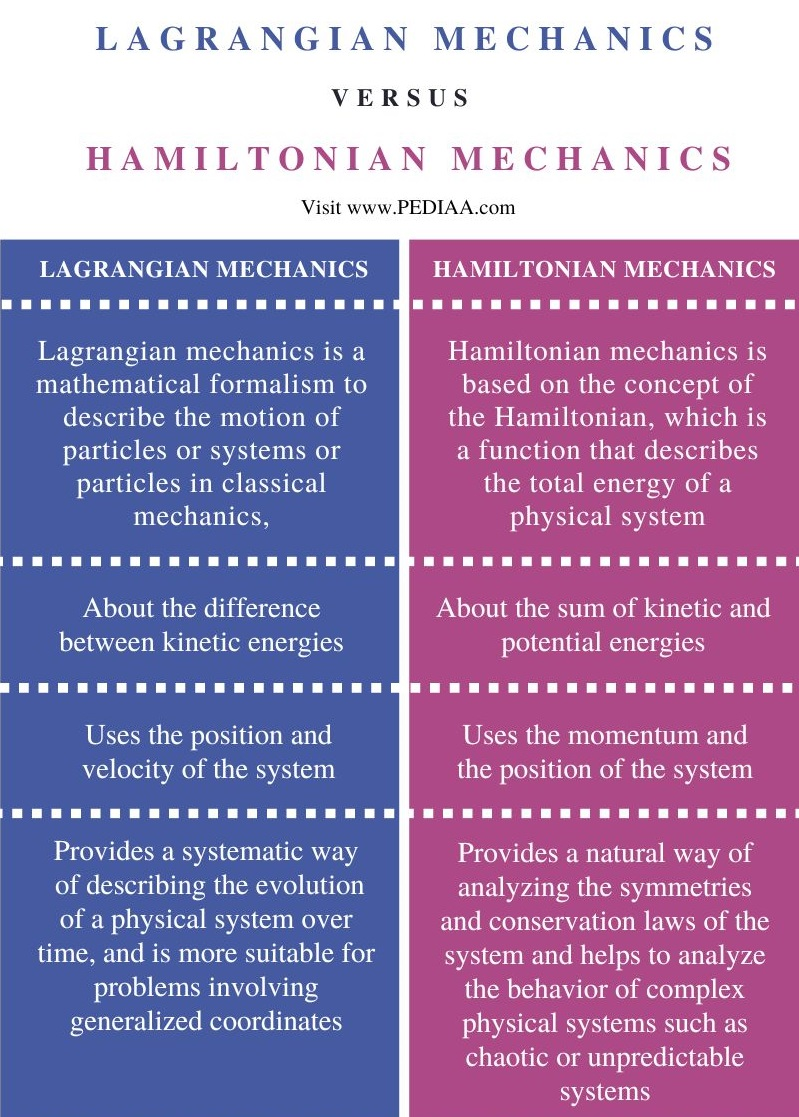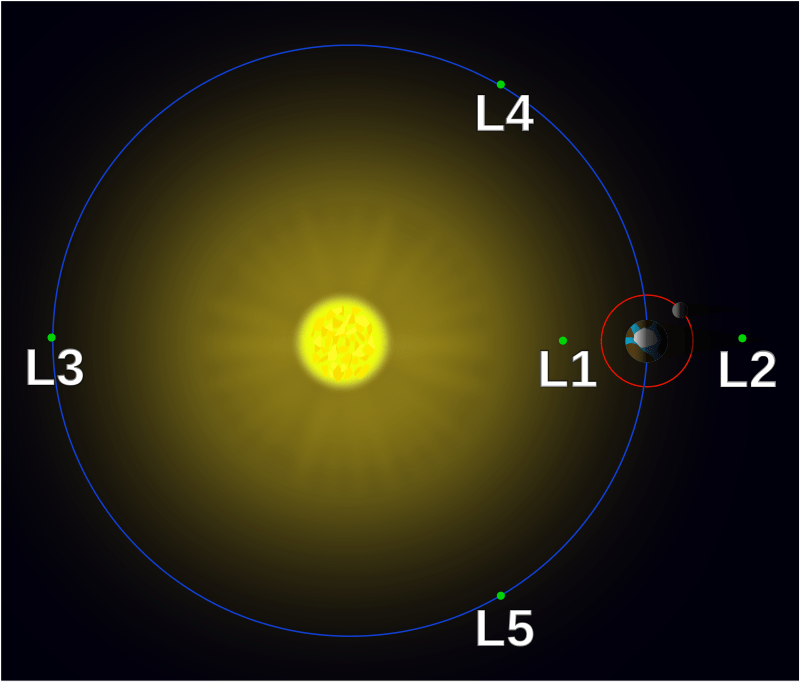# What is the Difference Between Lagrangian and Hamiltonian Mechanics

The main difference between Lagrangian and Hamiltonian mechanics is that Lagrangian mechanics is about the difference between kinetic energies, whereas Hamiltonian mechanics is about the sum of kinetic and potential energies.

Lagrangian mechanics and Hamiltonian mechanics are two different formulations of classical mechanics that describe the motion of particles or systems of particles.

### Key Areas Covered

1. What is Lagrangian Mechanics
2. What is Hamiltonian Mechanics
3. Similarities Between Lagrangian and Hamiltonian Mechanics
– Outline of Common Features
4. Difference Between Lagrangian and Hamiltonian Mechanics
– Comparison of Key Differences

### Key Terms

Lagrangian Mechanics, Hamiltonian Mechanics## What is Lagrangian Mechanics

Lagrangian mechanics is a mathematical formalism used to describe the motion of particles or systems or particles in classical mechanics. The Lagrangian formulation is based on the principle of least action, which states that the motion of a particle is such that the action defined as the integral of the Lagrangian over time is minimized.

Moreover, in Lagrangian mechanics, the fundamental quantity that describes the physics of a system is the Lagrangian, which is a function of the particle’s position, velocity, and time. The Lagrangian is defined as the difference between kinetic energy and potential energy of the system. The equations of motion obtained from the Lagrangian formulation are called the Euler-Langrange equations.There are many advantages of the Lagrangian formulation of mechanics over the Newtonian approach. First, it enables us to describe the motion of a system with a single scalar function, the Langrangian, instead of relying on multiple vector equations. Secondly, it provides a systematic way to incorporate constraints into the equations of motion. The Lagrangian approach allows us to take these constraints into account by the introduction of additional terms in the Lagrangian.

Besides, another advantage of the Lagrangian formulation is that it is more suitable for problems involving generalized coordinates. The Lagrangian approach can also be extended to systems with multiple particles. In addition, it can be extended to include external forces and non-conservative forces such as friction or air resistance. In this case, the Lagrangian undergoes modification to include these forces, and the resulting equations of motion take into account their effects on the system.

One important application of Lagrangian mechanics is in the study of classical field theory, where the Lagrangian helps to describe the behavior of fields such as the electromagnetic field or the gravitational field. It is also useful in the fields of engineering, physics, celestial mechanics, and quantum mechanics. Robotics, structural analysis, and control theory. The Lagrangian approach helps in the design of control systems for robots and other mechanical devices, as well as in the analysis of the motion of satellites and spacecraft.

## What is Hamiltonian Mechanics

Hamiltonian mechanics is based on the concept of energy. It is based on the concept of the Hamiltonian, which is a function that describes the total energy of a physical system. Hamiltonian is a combination of the system’s kinetic energy and potential energy and is typically denoted by the symbol H.

Mathematically the Hamiltonian is defined as,

H(p, q) = T(p) + V(q)

Where p and q are vectors that represent the system’s position and momentum, respectively. T(p) is the kinetic energy of the system, and V(q) is the potential energy.One key feature of Hamiltonian mechanics is that it provides a systematic way of analyzing the symmetries and conservation laws of physical systems. Hamiltonian equations have a number of important symmetries that reflect the underlying structure of the physical system.

One of the main advantages of Hamiltonian mechanics is that it provides a natural way of describing the evolution of a physical system over time. In particular, the Hamiltonian equation of motion gives a set of differential equations that describe the time evolution of the position and momentum of the system. We call these equations Hamiltonian equations.

Hamiltonian mechanics are useful in the fields of celestial mechanics, quantum mechanics, statistical mechanics, and fluid mechanics.

## Similarities Between Lagrangian and Hamiltonian Mechanics

• Both frameworks are based on the principle of least action.
• Both help in the fields of celestial mechanics and quantum mechanics.

## Difference Between Lagrangian and Hamiltonian Mechanics

### Definition

Lagrangian mechanics is a mathematical formalism to describe the motion of particles or systems or particles in classical mechanics, while Hamiltonian mechanics is based on the concept of the Hamiltonian, which is a function that describes the total energy of a physical system.

### Basics

Lagrangian mechanics is about the difference between kinetic energies, whereas Hamiltonian mechanics is about the sum of kinetic and potential energies.

### Variables that Describe the Physical System

Lagrangian uses the position and velocity of the system, whereas Hamiltonian mechanics uses the momentum and the position of the system.

Moreover, Lagrangian mechanics provides a systematic way of describing the evolution of a physical system over time, and it is more suitable for problems involving generalized coordinates. On the other hand, Hamiltonian mechanics provides a natural way of analyzing the symmetries and conservation laws of the system and helps to analyze the behavior of complex physical systems such as chaotic or unpredictable systems.

### Applications

Lagrangian mechanics are used in the fields of engineering, physics, celestial mechanics, and quantum mechanics. Robotics, structural analysis, and control theory, whereas Hamiltonian mechanics are used in the fields of celestial mechanics, quantum mechanics, statistical mechanics and fluid mechanics.

### Conclusion

Lagrangian mechanics and Hamiltonian mechanics are two different formulations of classical mechanics that describe the motion of particles or systems of particles. The main difference between Lagrangian and Hamiltonian mechanics is that Lagrangian mechanics is about the difference between kinetic energies, whereas Hamiltonian mechanics is about the sum of kinetic and potential energies.

##### Reference:

1. “Lagrangian Mechanics.” Brilliant.org
2. “Hamiltonian Mechanics.” Wikipedia. Wikipedia Foundation.

##### Image Courtesy:

1. “Lagrange points simple” By Xander89 – File:Lagrange_points2.SVG (CC BY 3.0) via Commons Wikimedia
2. “Hamiltonian Optics-Refraction” By Own work – Own work (CC BY-SA 3.0) via Commons Wikimedia### About the Author: Hasini A

Hasini is a graduate of Applied Science with a strong background in forestry, environmental science, chemistry, and management science. She is an amateur photographer with a keen interest in exploring the wonders of nature and science.